SSC BOARD PAPERS IMPORTANT TOPICS COVERED FOR BOARD EXAM 2024

### Practice Set 5.5, Chapter 5 - Quadrilaterals Mathematics Part II Solutions for Class 9 Math

###### Practice Set 5.5
Question 1.

In figure 5.38, points X, Y, Z are the midpoints of side AB, side BC and side AC of ΔABC respectively. AB = 5 cm, AC = 9 cm and BC = 11 cm. Find the length of XY, YZ, XZ.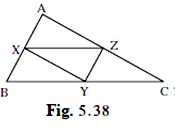Given X , Y and Z is the mid-point of AB, BC and AC.

Length of AB = 5 cm

So length of ZY = 1/2 × AB =1/2 × 5=2.5 cm (line joining mid-point of two sides of a triangle is parallel of the third side and is half of it)

Similarly, XZ = 1/2 × BC = 1/2 × 11= 5.5cm

Similarly, XY = 1/2 × AC = 1/2 × 9 = 4.5cm

Question 2.

In figure 5.39, 􀀍PQRS and 􀀍MNRL are rectangles. If point M is the midpoint of side PR then prove that,

i. SL = LR. Ii. LN = 1/2SQ.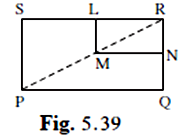The two rectangle PQRS and MNRL

In Δ PSR,

∠ PSR = ∠ MLR = 90°

∴ ML ∥ SP when SL is the transversal

M is the midpoint of PR (given)

By mid-point theorem a parallel line drawn from a mid-point of a side of a Δ meets at the Mid-point of the opposite side.

Hence L is the mid-point of SR

⇒ SL= LR

Similarly if we construct a line from L which is parallel to SR

This gives N is the midpoint of QR

Hence LN∥ SQ and L and N are mis points of SR and QR respectively

And LN = 1/2 SQ (mid-point theorem)

Question 3.

In figure 5.40, ΔABC is an equilateral triangle. Points F,D and E are midpoints of side AB, side BC, side AC respectively. Show that ΔEFD is an equilateral triangle.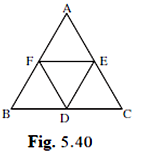Given F, D and E are mid-point of AB, BC and AC of the equilateral ΔABC ∴ AB =BC = AC

So by mid-point theorem

Line joining mid-points of two sides of a triangle is 1/2 of the parallel third side.

∴ FE = 1/2 BC =

Similarly, DE = 1/2 AB

And FD = 1/2 AC

But AB =BC = AC

⇒ 1/2 AB = 1/2 BC = 1/2 AC

⇒ DE = FD = FE

Since all the sides are equal ΔDEF is a equilateral triangle.

Question 4.

In figure 5.41, seg PD is a median of ΔPQR, Point T is the midpoint of seg PD. Produced QT intersects PR at M. Show that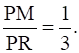[Hint : draw DN || QM.]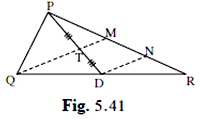PD is median so QD = DR (median divides the side opposite to vertex into equal halves)

T is mid-point of PD

⇒ PT = TD

In ΔPDN

T is mid-point and is ∥ to TM (by construction)

⇒TM is mid-point of PN

PM =MN……………….1

Similarly in ΔQMR

QM ∥ DN (construction)

D is mid –point of QR

⇒ MN = NR…………………..2

From 1 and 2

PM = MN = NR

Or PM = 1/3 PR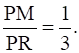hence proved